• Data Science
• James Le
• NOV 15, 2017

# The Ten Statistical Techniques Data Scientists Need to Master

Ready to learn Data Science? Browse courses like Data Science Training and Certification developed by industry thought leaders and Experfy in Harvard Innovation Lab.

Regardless of where you stand on the matter of Data Science sexiness, it’s simply impossible to ignore the continuing importance of data, and our ability to analyze, organize, and contextualize it. Drawing on their vast stores of employment data and employee feedback, Glassdoor ranked Data Scientist #1 in their 25 Best Jobs in America list. So the role is here to stay, but unquestionably, the specifics of what a Data Scientist does will evolve. With technologies like Machine Learning becoming ever-more common place, and emerging fields like Deep Learning gaining significant traction amongst researchers and engineers — and the companies that hire them — Data Scientists continue to ride the crest of an incredible wave of innovation and technological progress.

While having a strong coding ability is important, data science isn’t all about software engineering (in fact, have a good familiarity with Python and you’re good to go). Data scientists live at the intersection of coding, statistics, and critical thinking. As Josh Wills put it, “data scientist is a person who is better at statistics than any programmer and better at programming than any statistician.” I personally know too many software engineers looking to transition into data scientist and blindly utilizing machine learning frameworks such as TensorFlow or Apache Spark to their data without a thorough understanding of statistical theories behind them. So comes the study of statistical learning, a theoretical framework for machine learning drawing from the fields of statistics and functional analysis.

Why study Statistical Learning? It is important to understand the ideas behind the various techniques, in order to know how and when to use them. One has to understand the simpler methods first, in order to grasp the more sophisticated ones. It is important to accurately assess the performance of a method, to know how well or how badly it is working. Additionally, this is an exciting research area, having important applications in science, industry, and finance. Ultimately, statistical learning is a fundamental ingredient in the training of a modern data scientist. Examples of Statistical Learning problems include:

• Identify the risk factors for prostate cancer.
• Classify a recorded phoneme based on a log-periodogram.
• Predict whether someone will have a heart attack on the basis of demographic, diet and clinical measurements.
• Customize an email spam detection system.
• Identify the numbers in a handwritten zip code.
• Classify a tissue sample into one of several cancer classes.
• Establish the relationship between salary and demographic variables in population survey data.

In my last semester in college, I did an Independent Study on Data Mining. The class covers expansive materials coming from 3 books: Intro to Statistical Learning (Hastie, Tibshirani, Witten, James), Doing Bayesian Data Analysis(Kruschke), and Time Series Analysis and Applications (Shumway, Stoffer). We did a lot of exercises on Bayesian Analysis, Markov Chain Monte Carlo, Hierarchical Modeling, Supervised and Unsupervised Learning. This experience deepens my interest in the Data Mining academic field and convinces me to specialize further in it. Recently, I completed the Statistical Learning online course on Stanford Lagunita, which covers all the material in the Intro to Statistical Learning book I read in my Independent Study. Now being exposed to the content twice, I want to share the 10 statistical techniques from the book that I believe any data scientists should learn to be more effective in handling big datasets.

Before moving on with these 10 techniques, I want to differentiate between statistical learning and machine learning. I wrote one of the most popular Medium posts on machine learning before, so I am confident I have the expertise to justify these differences:

• Machine learning arose as a subfield of Artificial Intelligence.
• Statistical learning arose as a subfield of Statistics.
• Machine learning has a greater emphasis on large scale applications and prediction accuracy.
• Statistical learning emphasizes models and their interpretability, and precision and uncertainty.
• But the distinction has become and more blurred, and there is a great deal of “cross-fertilization.”
• Machine learning has the upper hand in Marketing!

## 1 — Linear Regression:

In statistics, linear regression is a method to predict a target variable by fitting the best linear relationship between the dependent and independent variable. The best fit is done by making sure that the sum of all the distances between the shape and the actual observations at each point is as small as possible. The fit of the shape is “best” in the sense that no other position would produce less error given the choice of shape. 2 major types of linear regression are Simple Linear Regression and Multiple Linear Regression. Simple Linear Regressionuses a single independent variable to predict a dependent variable by fitting a best linear relationship. Multiple Linear Regression uses more than one independent variable to predict a dependent variable by fitting a best linear relationship.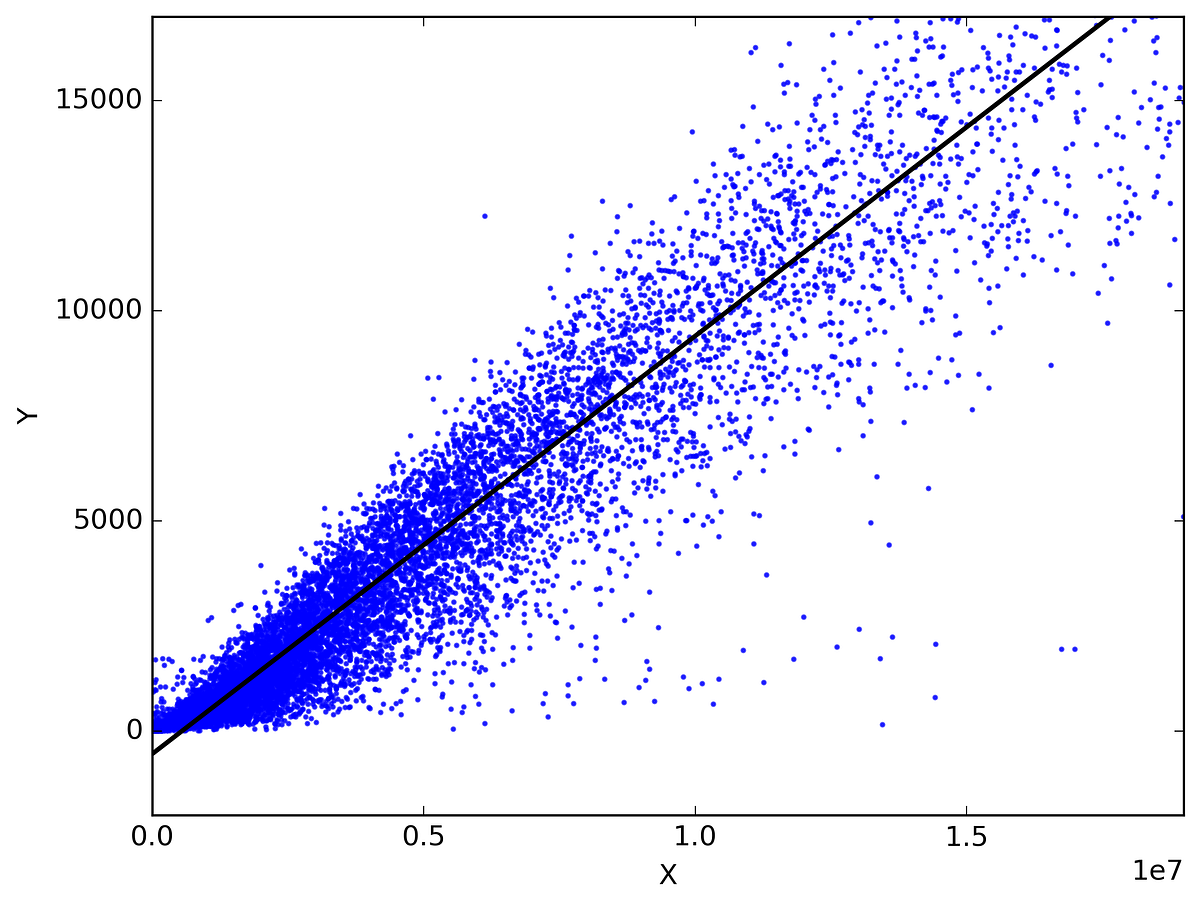Pick any 2 things that you use in your daily life and that are related. Like, I have data of my monthly spending, monthly income and the number of trips per month for the last 3 years. Now I need to answer the following questions:

• What will be my monthly spending for next year?
• Which factor (monthly income or number of trips per month) is more important in deciding my monthly spending?
• How monthly income and trips per month are correlated with monthly spending?

## 2 — Classification:

Classification is a data mining technique that assigns categories to a collection of data in order to aid in more accurate predictions and analysis. Also sometimes called a Decision Tree, classification is one of several methods intended to make the analysis of very large datasets effective. 2 major Classification techniques stand out: Logistic Regression and Discriminant Analysis.

Logistic Regression is the appropriate regression analysis to conduct when the dependent variable is dichotomous (binary). Like all regression analyses, the logistic regression is a predictive analysis. Logistic regression is used to describe data and to explain the relationship between one dependent binary variable and one or more nominal, ordinal, interval or ratio-level independent variables. Types of questions that a logistic regression can examine:

• How does the probability of getting lung cancer (Yes vs No) change for every additional pound of overweight and for every pack of cigarettes smoked per day?
• Do body weight calorie intake, fat intake, and participant age have an influence on heart attacks (Yes vs No)?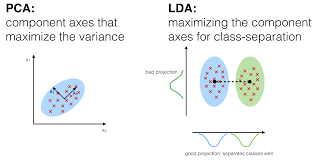In Discriminant Analysis, 2 or more groups or clusters or populations are known a priori and 1 or more new observations are classified into 1 of the known populations based on the measured characteristics. Discriminant analysis models the distribution of the predictors X separately in each of the response classes, and then uses Bayes’ theorem to flip these around into estimates for the probability of the response category given the value of X. Such models can either be linear or quadratic.

• Linear Discriminant Analysis computes “discriminant scores” for each observation to classify what response variable class it is in. These scores are obtained by finding linear combinations of the independent variables. It assumes that the observations within each class are drawn from a multivariate Gaussian distribution and the covariance of the predictor variables are common across all k levels of the response variable Y.
• Quadratic Discriminant Analysis provides an alternative approach. Like LDA, QDA assumes that the observations from each class of Y are drawn from a Gaussian distribution. However, unlike LDA, QDA assumes that each class has its own covariance matrix. In other words, the predictor variables are not assumed to have common variance across each of the k levels in Y.

## 3 — Resampling Methods:

Resampling is the method that consists of drawing repeated samples from the original data samples. It is a non-parametric method of statistical inference. In other words, the method of resampling does not involve the utilization of the generic distribution tables in order to compute approximate p probability values.

Resampling generates a unique sampling distribution on the basis of the actual data. It uses experimental methods, rather than analytical methods, to generate the unique sampling distribution. It yields unbiased estimates as it is based on the unbiased samples of all the possible results of the data studied by the researcher. In order to understand the concept of resampling, you should understand the terms Bootstrapping and Cross-Validation: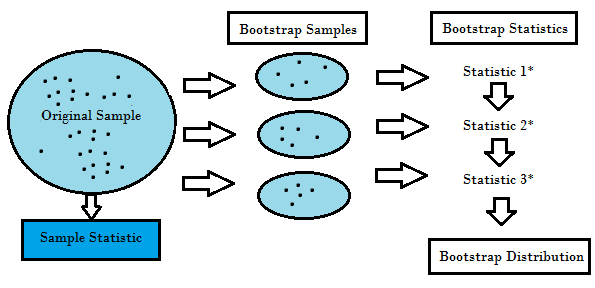• Bootstrapping is a technique that helps in many situations like validation of a predictive model performance, ensemble methods, estimation of bias and variance of the model. It works by sampling with replacement from the original data, and take the “not chosen” data points as test cases. We can make this several times and calculate the average score as estimation of our model performance.
• On the other hand, cross validation is a technique for validating the model performance, and it’s done by split the training data into k parts. We take the k — 1 parts as our training set and use the “held out” part as our test set. We repeat that k times differently. Finally, we take the average of the k scores as our performance estimation.

Usually for linear models, ordinary least squares is the major criteria to be considered to fit them into the data. The next 3 methods are the alternative approaches that can provide better prediction accuracy and model interpretability for fitting linear models.

## 4 — Subset Selection:

This approach identifies a subset of the p predictors that we believe to be related to the response. We then fit a model using the least squares of the subset features.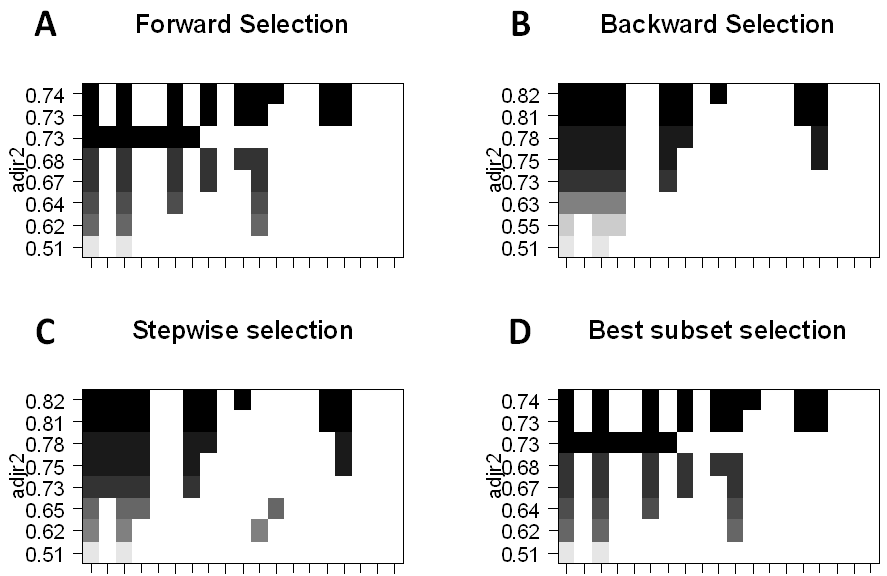• Best-Subset Selection: Here we fit a separate OLS regression for each possible combination of the p predictors and then look at the resulting model fits. The algorithm is broken up into 2 stages: (1) Fit all models that contain k predictors, where k is the max length of the models, (2) Select a single model using cross-validated prediction error. It is important to use testing or validation error, and not training error to assess model fit because RSS and R² monotonically increase with more variables. The best approach is to cross-validate and choose the model with the highest R² and lowest RSS on testing error estimates.
• Forward Stepwise Selection considers a much smaller subset of ppredictors. It begins with a model containing no predictors, then adds predictors to the model, one at a time until all of the predictors are in the model. The order of the variables being added is the variable, which gives the greatest addition improvement to the fit, until no more variables improve model fit using cross-validated prediction error.
• Backward Stepwise Selection begins will all p predictors in the model, then iteratively removes the least useful predictor one at a time.
• Hybrid Methods follows the forward stepwise approach, however, after adding each new variable, the method may also remove variables that do not contribute to the model fit.

## 5 — Shrinkage:

This approach fits a model involving all p predictors, however, the estimated coefficients are shrunken towards zero relative to the least squares estimates. This shrinkage, aka regularization has the effect of reducing variance. Depending on what type of shrinkage is performed, some of the coefficients may be estimated to be exactly zero. Thus this method also performs variable selection. The two best-known techniques for shrinking the coefficient estimates towards zero are the ridge regression and the lasso.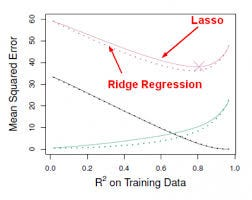• Ridge regression is similar to least squares except that the coefficients are estimated by minimizing a slightly different quantity. Ridge regression, like OLS, seeks coefficient estimates that reduce RSS, however they also have a shrinkage penalty when the coefficients come closer to zero. This penalty has the effect of shrinking the coefficient estimates towards zero. Without going into the math, it is useful to know that ridge regression shrinks the features with the smallest column space variance. Like in prinicipal component analysis, ridge regression projects the data into ddirectional space and then shrinks the coefficients of the low-variance components more than the high variance components, which are equivalent to the largest and smallest principal components.
• Ridge regression had at least one disadvantage; it includes all p predictors in the final model. The penalty term will set many of them close to zero, but never exactly to zero. This isn’t generally a problem for prediction accuracy, but it can make the model more difficult to interpret the results. Lasso overcomes this disadvantage and is capable of forcing some of the coefficients to zero granted that s is small enough. Since s = 1 results in regular OLS regression, as s approaches 0 the coefficients shrink towards zero. Thus, Lasso regression also performs variable selection.

## 6 — Dimension Reduction:

Dimension reduction reduces the problem of estimating p + 1 coefficients to the simple problem of M + 1 coefficients, where M < p. This is attained by computing M different linear combinations, or projections, of the variables. Then these M projections are used as predictors to fit a linear regression model by least squares. 2 approaches for this task are principal component regression and partial least squares.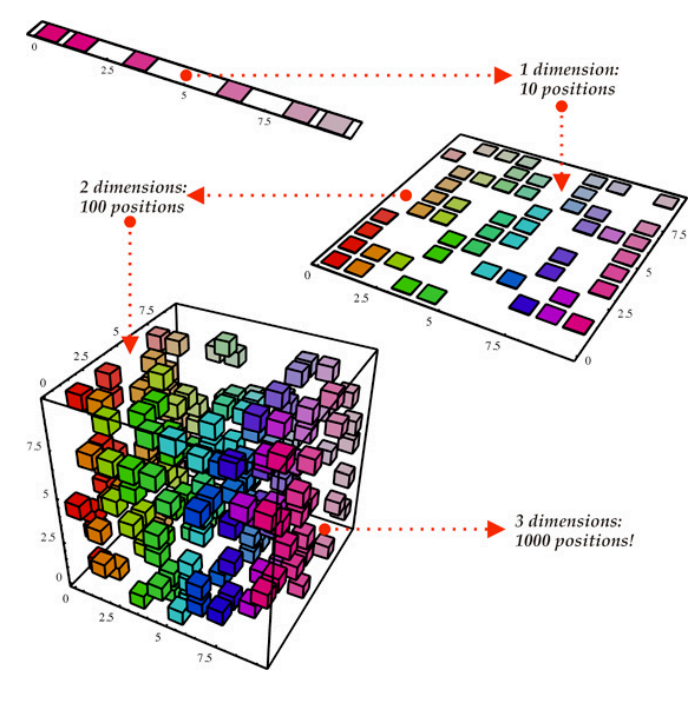• One can describe Principal Components Regression as an approach for deriving a low-dimensional set of features from a large set of variables. The firstprincipal component direction of the data is along which the observations vary the most. In other words, the first PC is a line that fits as close as possible to the data. One can fit p distinct principal components. The second PC is a linear combination of the variables that is uncorrelated with the first PC, and has the largest variance subject to this constraint. The idea is that the principal components capture the most variance in the data using linear combinations of the data in subsequently orthogonal directions. In this way, we can also combine the effects of correlated variables to get more information out of the available data, whereas in regular least squares we would have to discard one of the correlated variables.
• The PCR method that we described above involves identifying linear combinations of X that best represent the predictors. These combinations (directions) are identified in an unsupervised way, since the response Y is not used to help determine the principal component directions. That is, the response Y does not supervise the identification of the principal components, thus there is no guarantee that the directions that best explain the predictors also are the best for predicting the response (even though that is often assumed). Partial least squares (PLS) are a supervisedalternative to PCR. Like PCR, PLS is a dimension reduction method, which first identifies a new smaller set of features that are linear combinations of the original features, then fits a linear model via least squares to the new M features. Yet, unlike PCR, PLS makes use of the response variable in order to identify the new features.

## 7 — Nonlinear Models:

In statistics, nonlinear regression is a form of regression analysis in which observational data are modeled by a function which is a nonlinear combination of the model parameters and depends on one or more independent variables. The data are fitted by a method of successive approximations. Below are a couple of important techniques to deal with nonlinear models:

• A function on the real numbers is called a step function if it can be written as a finite linear combination of indicator functions of intervals. Informally speaking, a step function is a piecewise constant function having only finitely many pieces.
• piecewise function is a function which is defined by multiple sub-functions, each sub-function applying to a certain interval of the main function’s domain. Piecewise is actually a way of expressing the function, rather than a characteristic of the function itself, but with additional qualification, it can describe the nature of the function. For example, a piecewise polynomial function is a function that is a polynomial on each of its sub-domains, but possibly a different one on each.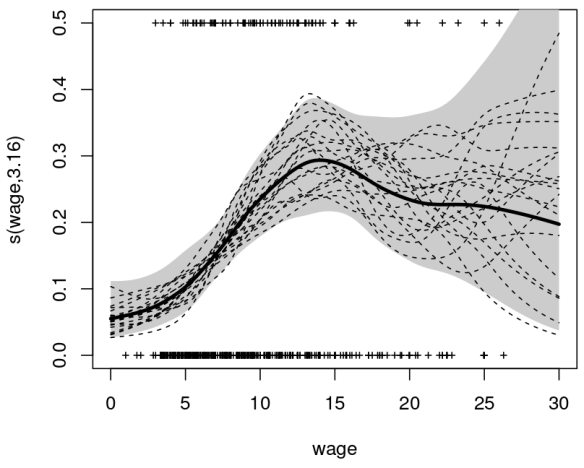• spline is a special function defined piecewise by polynomials. In computer graphics, spline refers to a piecewise polynomial parametric curve. Splines are popular curves because of the simplicity of their construction, their ease and accuracy of evaluation, and their capacity to approximate complex shapes through curve fitting and interactive curve design.
• generalized additive modelis a generalized linear model in which the linear predictor depends linearly on unknown smooth functions of some predictor variables, and interest focuses on inference about these smooth functions.

## 8 — Tree-Based Methods:

Tree-based methods can be used for both regression and classification problems. These involve stratifying or segmenting the predictor space into a number of simple regions. Since the set of splitting rules used to segment the predictor space can be summarized in a tree, these types of approaches are known as decision-tree methods. The methods below grow multiple trees which are then combined to yield a single consensus prediction.

• Bagging is the way decrease the variance of your prediction by generating additional data for training from your original dataset using combinations with repetitions to produce multistep of the same carnality/size as your original data. By increasing the size of your training set you can’t improve the model predictive force, but just decrease the variance, narrowly tuning the prediction to expected outcome.
• Boosting is an approach to calculate the output using several different models and then average the result using a weighted average approach. By combining the advantages and pitfalls of these approaches by varying your weighting formula you can come up with a good predictive force for a wider range of input data, using different narrowly tuned models.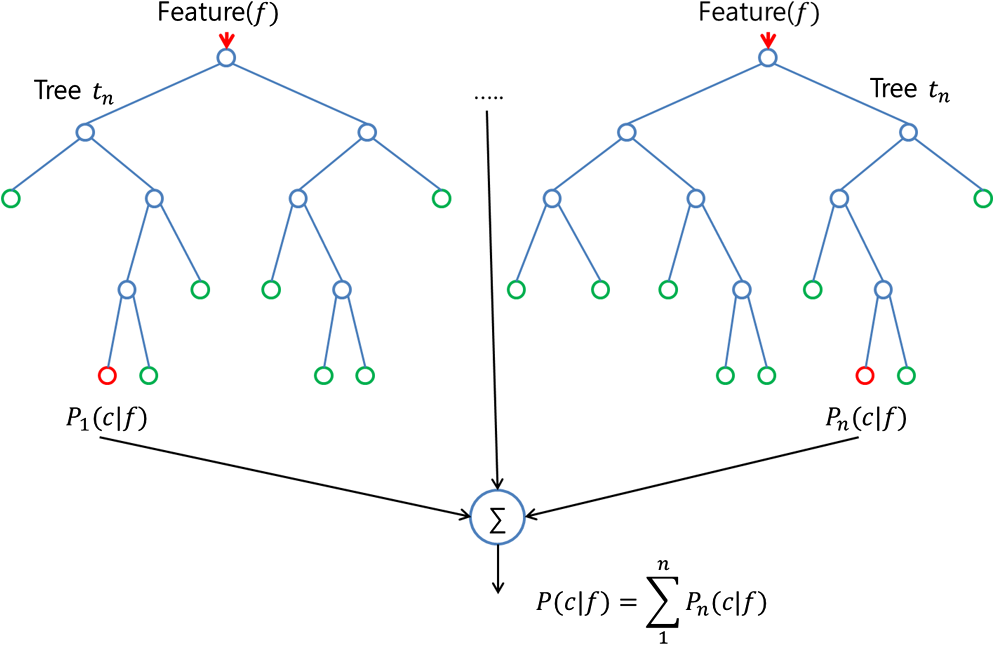• The random forest algorithm is actually very similar to bagging. Also here, you draw random bootstrap samples of your training set. However, in addition to the bootstrap samples, you also draw a random subset of features for training the individual trees; in bagging, you give each tree the full set of features. Due to the random feature selection, you make the trees more independent of each other compared to regular bagging, which often results in better predictive performance (due to better variance-bias trade-offs) and it’s also faster, because each tree learns only from a subset of features.

## 9 — Support Vector Machines: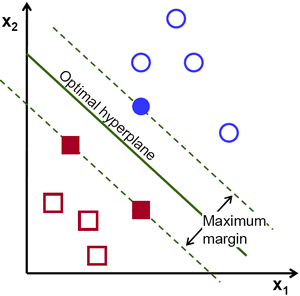SVM is a classification technique that is listed under supervised learning models in Machine Learning. In layman’s terms, it involves finding the hyperplane (line in 2D, plane in 3D and hyperplane in higher dimensions. More formally, a hyperplane is n-1 dimensional subspace of an n-dimensional space) that best separates two classes of points with the maximum margin. Essentially, it is a constrained optimization problem where the margin is maximized subject to the constraint that it perfectly classifies the data (hard margin).

The data points that kind of “support” this hyperplane on either sides are called the “support vectors”. In the above picture, the filled blue circle and the two filled squares are the support vectors. For cases where the two classes of data are not linearly separable, the points are projected to an exploded (higher dimensional) space where linear separation may be possible. A problem involving multiple classes can be broken down into multiple one-versus-one or one-versus-rest binary classification problems.

10 — Unsupervised Learning:

So far, we only have discussed supervised learning techniques, in which the groups are known and the experience provided to the algorithm is the relationship between actual entities and the group they belong to. Another set of techniques can be used when the groups (categories) of data are not known. They are called unsupervised as it is left on the learning algorithm to figure out patterns in the data provided. Clustering is an example of unsupervised learning in which different data sets are clustered into groups of closely related items. Below is the list of most widely used unsupervised learning algorithms: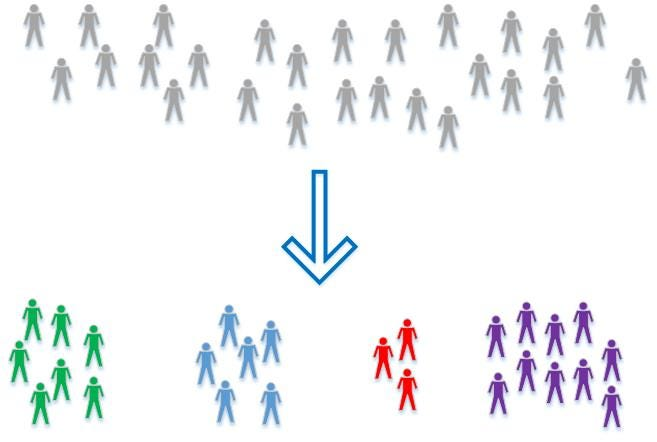• Principal Component Analysis helps in producing low dimensional representation of the dataset by identifying a set of linear combination of features which have maximum variance and are mutually un-correlated. This linear dimensionality technique could be helpful in understanding latent interaction between the variable in an unsupervised setting.
• k-Means clustering: partitions data into k distinct clusters based on distance to the centroid of a cluster.
• Hierarchical clustering: builds a multilevel hierarchy of clusters by creating a cluster tree.

This was a basic run-down of some basic statistical techniques that can help a data science program manager and or executive have a better understanding of what is running underneath the hood of their data science teams. Truthfully, some data science teams purely run algorithms through python and R libraries. Most of them don’t even have to think about the math that is underlying. However, being able to understand the basics of statistical analysis gives your teams a better approach. Have insight into the smallest parts allows for easier manipulation and abstraction. I hope this basic data science statistical guide gives you a decent understanding!

P.S: You can get all the lecture slides and RStudio sessions from my GitHub source code here. Thanks for the overwhelming response!

Originally published at Towards Data Science